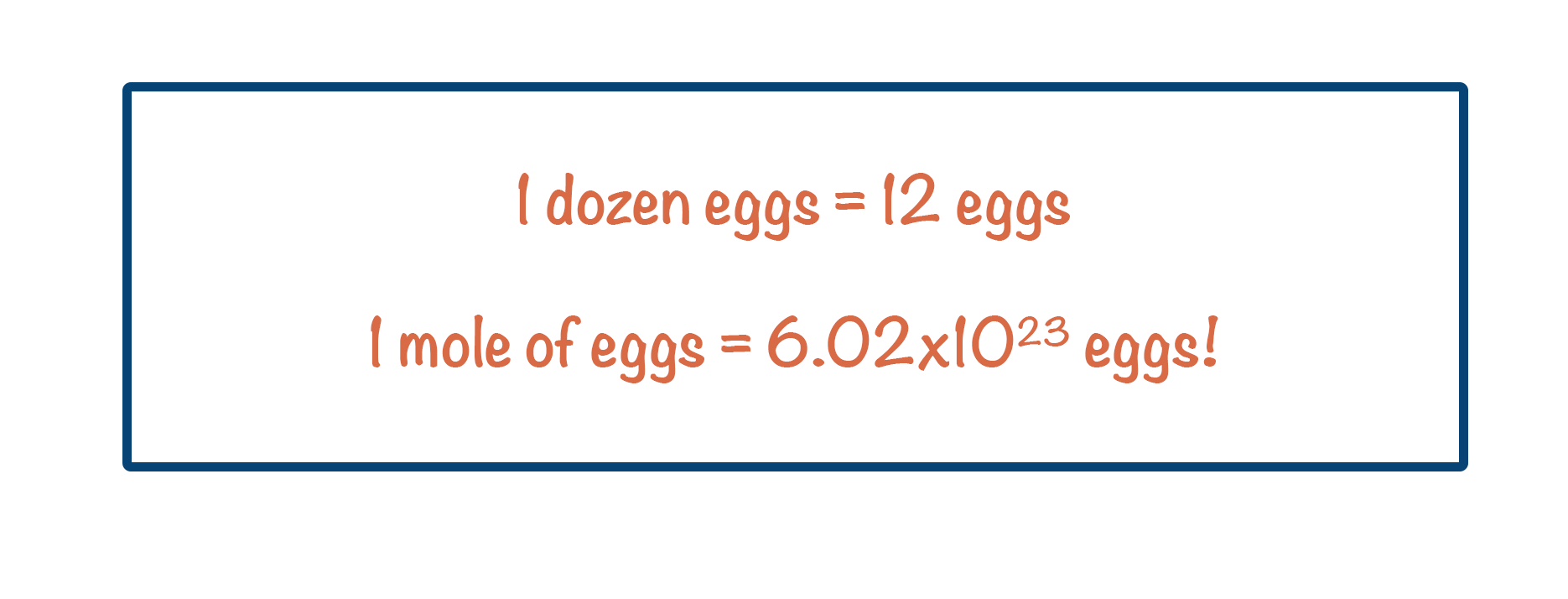Video Tutorial The Mole

Quick Notes The Mole

• Atoms and particles are very small, so referring to actual number of atoms in a sample isn’t very practical.
• The mole is a unit used to refer to an amount of atoms or particles.
• Just like one dozen = 12 of something, one mole = 6.022 x 1023 of something.
• The number one mole refers to is known as Avogadro’s constant.

Full Notes The Mole

In chemistry there are two real ways of measuring how much matter (‘something’) we have – mass and volume.

Atoms are very small. This means, even for a small sample, a substance will have a lot of atoms in it. So many in fact, it is not realistic to refer to the whole number of atoms or particles in the sample. Instead, we use a unit called ‘the mole’. A mole is just a way of saying how many particles of something you have.

Just like 1 dozen = 12 of something; 1 mole = 6.02 x 1023 of something.If you have two dozen atoms, you know that you have twenty-four atoms (2 x 12 = 24). If you have two moles worth of atoms in a sample, it simply means you have 1.25 x 1024 atoms of that sample (2 x 6.02 x 1023).